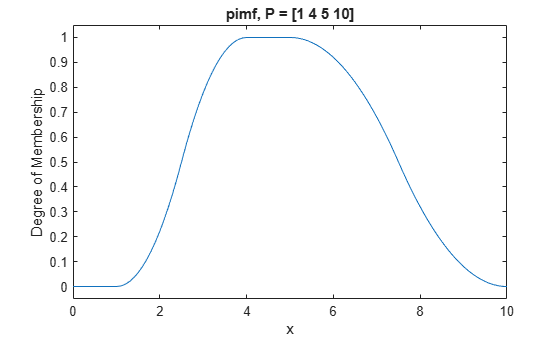# pimf

Pi-shaped membership function

## Syntax

``y = pimf(x,params)``

## Description

This function computes fuzzy membership values using a spline-based pi-shaped membership function. You can also compute this membership function using a `fismf` object. For more information, see fismf Object.

This membership function is related to the `smf` and `zmf` membership functions.

example

````y = pimf(x,params)` returns fuzzy membership values computed using a spline-based pi-shaped membership function. This membership function is the product of an `smf` function and a `zmf` function, and is given by:To specify the a, b, c, and d parameters, use `params`.Membership values are computed for each input value in `x`.```

## Examples

collapse all

```x = 0:0.1:10; y = pimf(x,[1 4 5 10]); plot(x,y) xlabel('pimf, P = [1 4 5 10]') ylim([-0.05 1.05])```## Input Arguments

collapse all

Input values for which to compute membership values, specified as a scalar or vector.

Membership function parameters, specified as the vector [a b c d]. Parameters a and d define the feet of the membership function, and b and c define its shoulders.

## Output Arguments

collapse all

Membership value returned as a scalar or a vector. The dimensions of `y` match the dimensions of `x`. Each element of `y` is the membership value computed for the corresponding element of `x`.

## Alternative Functionality

### `fismf` Object

You can create and evaluate a `fismf` object that implements the `pimf` membership function.

```mf = fismf("pimf",P); Y = evalmf(mf,X);```

Here, `X`, `P`, and `Y` correspond to the `x`, `params`, and `y` arguments of `pimf`, respectively.